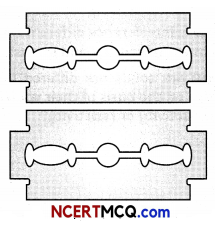Students can access the CBSE Sample Papers for Class 12 Physics with Solutions and marking scheme Term 2 Set 10 will help students in understanding the difficulty level of the exam.

## CBSE Sample Papers for Class 12 Physics Standard Term 2 Set 10 for Practice

Time Allowed: 2 Hours
Maximum Marks: 40

General Instructions:

• There are 12 questions in all. All questions are compulsory.
• This question paper has three sections: Section A, Section B and Section C.
• Section A contains three questions of two marks each, Section B contains eight questions of three marks each, Section C contains one case study-based question of five marks.
• There is no overall choice. However, an internal choice has been provided in one question of two marks and two questions of three marks. You have to attempt only one of the chokes in such questions.
• You may use log tables if necessary but use of calculator is not allowed.

SECTION – A
(Section A contains 3 questions of 2 marks each.)

Question 1.
Clarify the difference between Rutherford’s atomic model and Bohr’s atomic model justify your answer.
OR
What characteristic property of nuclear force explains the constancy of binding energy per nucleon (BE/A) in the range of mass number ‘A’ lying 30 < A < 170? (2)

Question 2.
How is interference different from diffraction in terms of sources and intensity? (2)Question 3.
How are solids are differed if we compare them on the basis of their values of electrical conductivity or resistivity? (2)

SECTION – B
(Section B consists of 8 questions of 3 marks each.)

Question 4.
The frequency band of a radio station is 6 MHz to 8 MHz. What is corresponding wavelength band? (3)

Question 5.
(A) de-Broglie wavelength associated with
macroscopic objects (car, cricket ball etc.) cannot be observed in daily life. Why?
(B) The wavelength of light from the spectral emission line of sodium is 590 nm. Find the kinetic energy at which the electron would have the same de Broglie wavelength. (3)
OR
(A) Name the series in hydrogen spectrum whose few lines lies in the visible region.
(B) Calculate the orbital period of the electron in the first excited state of hydrogen atom. (2)

Question 6.
Explain the formation of depletion layer and potential barrier in p-n junction. (3)

Question 7.
State the difference between Interference and Diffraction. (3)Question 8.
(A) What is refraction?
(B) An 2 cm tall object is placed on the axis of a convex lens of focal length 5 cm at a distance of 10 m from the optical center of the lens. Find the nature, position and size of the image formed. Which case of image formation by convex lenses is illustrated by this example?

Question 9.
Write Einstein’s photoelectric equation and point out any two characteristic properties of photons on which this equation is based. Briefly explain the three observed features which can be explained by this equation. (3)

Question 10.
Differentiate between n-type semiconduc¬tors and p type semiconductors. (3)

Question 11.
(A) What is the use of erecting lens in a terrestrial telescope? What is its magnification?
(B) The focal length of objective and eyepiece of an astronomical telescope are 20 cm and 5 cm respectively. If the final image is formed at a distance of 30 cm from the eye piece, then find the magnifying power of telescope? (3)
OR
(A) A concave lens of refractive index 1.5 is immersed in a medium of refractive index 1.65. What is the nature of the lens?
(B) A convex lens of focal length 20 cm is placed coaxially in contact with a concave lens of focal length 25 cm. Determine the power of the combination.SECTION – C
(Section C consists one case study-based question of 5 marks)

Question 12.
You can see the single slit diffraction pattern easily by using just a pair of blades and one clear glass electric bulb. In order to observe the diffraction, you have to hold the blades so that the edges are parallel and hence have a narrow slit between them. By keeping the slit parallel to the filament, right in front of the eye, you will be able to see a pattern. The filament plays the role of the first slit S.(A) A slit of width a is illuminated by white light. For red light (λ = 6500 Å). The first minima is obtained at θ = 30°. Then the value of a will be:
(i) 3250 Å
(ii) 6.5 × 10-4 mm
(iii) 1.24 microns
(iv) 2.6 × 10-4 cm

(B) A beam of light of λ = 600 nm from a distant source falls on a single slit 1 mm wide and the resulting diffraction pattern is observed on a screen 2 m away. The distance between first dark fringes on either side of the central bright fringe is:
(i) 1.2 cm
(ii) 1.2 mm
(iii) 2.4 cm
(iv) 2.4 mm

(C) The width of the diffraction band varies:
(i) Inversely as the wavelength
(ii) Directly as the width of the slit
(iii) Directly as the distance between the slit and the screen
(iv) Inversely as the size of the source from which the slit is illuminated.

(D) While both light and sound show wave character, diffraction is much harder to observe in light. This is because:
(i) Wavelength of light is far smaller
(ii) Waves of light are transverse
(iii) Speed of light is far greater
(iv) Light does not require any medium(E) In a diffraction pattern due to a single slit of width a, the first minimum is observed at an angle 30° when light of wavelength 5000 Å is incident on the slit. The first secondary maximum is observed at an angle of:
(i) sin-1 ($$\frac{1}{2}$$)
(ii) sin-1 ($$\frac{3}{4}$$)
(iii) sin-1 ($$\frac{1}{4}$$)
(iv) sin’-1 ($$\frac{2}{3}$$)